+

# Operations as Grouping Symbols

Author: Sophia Tutorial
##### Description:

Evaluate an expression with absolute value using order of operations.

(more)

Sophia’s self-paced online courses are a great way to save time and money as you earn credits eligible for transfer to many different colleges and universities.*

No credit card required

37 Sophia partners guarantee credit transfer.

299 Institutions have accepted or given pre-approval for credit transfer.

* The American Council on Education's College Credit Recommendation Service (ACE Credit®) has evaluated and recommended college credit for 32 of Sophia’s online courses. Many different colleges and universities consider ACE CREDIT recommendations in determining the applicability to their course and degree programs.

Tutorial
what's covered
1. Operations as Grouping Symbol
1. Fraction Bars
2. Absolute Value Bars

# 1. Operations as Grouping Symbols

The order of operations outlines an order we should follow to evaluate expressions with multiple operations. We often use the acronym PEMDAS to remember the order of operations:

term to know
PEMDAS
An acronym to remember the order of operations: parentheses, exponents, multiplication and division (from left to right), addition and subtraction (from left to right).

It is important to remember that the "parentheses" part of the order of operations includes grouping symbols, such as braces or brackets. There are several types of operations that act as grouping symbols. This means that they are operations, but need to be considered first in the order of operations, because they also enclose other expressions in parentheses, even though they might not be visible.

In this lesson, we will see three operations that act as grouping symbols: fraction bars, absolute value bars, and the radical symbol.

1a. Fraction Bars

If we have a fraction, the entire numerator and the entire denominator must be evaluated before we reduce the fraction. In these cases, we can simplify in both the numerator and denominator at the same time.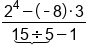Exponent in the numerator, divide in denominatorMultiply in the numerator, subtract in the denominatorAdd the opposite to simplify numerator, denominator is done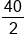Reduce, divide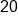Our Solution

big idea
A fraction bar groups numbers and operations in a numerator and denominator. Although a fraction bar represents division, it creates implied parentheses around everything on top and on the bottom. We must fully evaluate both the numerator and denominator of a fraction, and divide at the very end.

1b. Absolute Value Bars

Another type of grouping symbol that also has an operation with it is absolute value. When we have an absolute value, we will evaluate everything inside the absolute value bars, just as if it were a normal parentheses. Then once the inside is completed, we will take the absolute value, or distance from zero, to make sure the number is non-negative.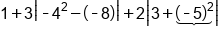Evaluate absolute first, exponents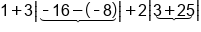Add inside absolute values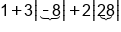Evaluate absolute values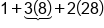Multiply left to right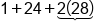Finish multiplying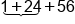Add left to right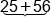Add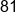Our Solution

big idea
Absolute value bars also act as a grouping symbol, and come first in the order of operations. If we see an expression inside absolute value bars, we must completely evaluate that expression, then take its absolute value, before moving on to the other operations according to the order of operations.

hint
The above example also illustrates an important point about exponents. Exponents are considered to be only on the number they are attached to. This means when we see -42, only the 4 is squared, giving us -(42) or -16. But when the negative is in parentheses, such as (-5)2, the negative is part of the number and is also squared, giving us a positive solution, 25.

Expressions can be underneath radical symbols, just like they can be in numerators, denominators, or within absolute value bars. When an expression is underneath a radical, this groups the expression together, and acts as a grouping symbol. This means we must fully evaluate the expression underneath the radical sign, before moving on to other operations in the order of operations. Let's take a look at an example: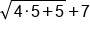The radical sign groups the expression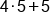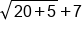Multiply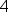by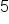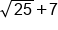Add 5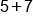Evaluate the square root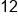Our Solution

summary
Grouping symbols fall under parentheses in PEMDAS, which means that when you're evaluating with the order of operations, you're going to start with grouping symbols first. Grouping symbols include fraction bars, absolute value signs, and radicals. For nested grouping symbols, where you have more than one grouping symbol inside of another one, you need to start by evaluating them from the inside out. Whatever your innermost grouping symbol is, start with that, and then work your way out.

Terms to Know
PEMDAS

An acronym to remember the order of operations: parentheses, exponents, multiplication and division, addition and subtraction.

Rating